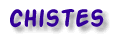``````
 Busca enCIENCIAnet ¿cómo buscar? Anécdotas Curiosidades Elementos Experimentos La Pregunta Archivo Textos Clásicos En la Cocina Citas Chistes Exámenes Libros Enlaces Más ...
The Student's Guide to Problem Solving

1. If at all possible, avoid reading the problem. Reading the problem only consumes time and causes confusion.

2. Extract the numbers from the problem in the order in which they appear. Be on the watch for numbers written in words.

3. If rule 2 yields three or more numbers, the best bet for getting the answer is to add them together.

4. If there are only two numbers which are approximately the same size, then subtraction should give the best results.

5. If there are only two numbers in the problem and one is much smaller than the other, then divide if it goes exactly, otherwise multiply.

6. If the problem seems like it calls for a formula, pick a formula that has enough letters to use all the numbers in the problem.

7. If the rules 1 - 6 don't seems to work, make one last desperate attempt. Take the set of numbers found by rule 2 and perform about 2 pages of random operations using these numbers. You should circle about five or six answers on each page just in case one of them happens to be the answer. You might get some partial credit for trying hard.

8. Never, never spend too much time solving problems. This set of rules will get you through even the longest assignments in no more than 10 minutes with very little thinking.

Joe Dodson, Mathematics Supervisor, Winston-Salem/Forsyth County Schools, North Carolina

Volver a la guía del estudiante